# Fundamentals of Engineering Economics

## Quiz 9 : Accounting for Depreciation and Income TaxesStudy FlashcardsLooking for Economics Homework Help?

## Quiz 9 :Accounting for Depreciation and Income Taxes

Question TypeA company purchased a drill press priced at $170,000 in year 0. The company additionally incurred$30,000 for site preparation and labor to install the machine. The drill press was classified as a seven-year MACRS class property. The company is considering selling the drill press for $70,000 at the end of year 4. Compute the book value at the end of year 4 that should be used in calculating the taxable gains. (a)$62,480 (b) $53,108 (c)$63,725 (d) $74,970 Free Multiple Choice Answer: Answer: It is given that the initial cost of the drill is$170,000 and its installation costs $30,000. Thus, the total cost of drill on which depreciation is applied is$200,000 ($170,000+$30,000). It is classified as a 7-year MACRS class property. The salvage value at the time of selling, that is, at the end of year-4, is $70,000. Depreciation rates (7-year MACRS)Table-1 shows the depreciation rates of 7-year MACRS class property for 4 years.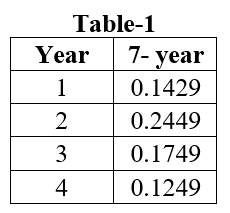Following is the way to calculate the total depreciation allowed:Thus, the total depreciation allowed is$125,030
(NOTE: For a MACRS property, if an asset is disposed during or before its specified period, the year of disposal is charged one-half of that year's annual depreciation amount )Following is the formula to calculate the book value of the machine:Thus, the book value at the end of year-4 is $74,970 The correct option is (D). Tags Choose question tagOmar Shipping Company bought a tugboat for$75,000 (year 0) and expected to use it for five years after which it will be sold for $12,000. Suppose the company estimates the following revenues and expenses from the tugboat investment for the first operating year: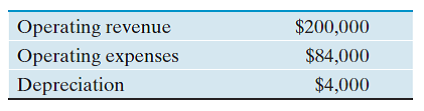If the company pays taxes at the rate of 30% on its taxable income, what is the net income during the first year? (a)$28,700 (b) $81,200 (c)$78,400 (d) $25,900 Free Multiple Choice Answer: Answer: Given information: • Price of tugboat is$75,000.
• Salvage value is $12,000 after 5 years. • Operating revenue is$200,000.
• Operating expense is $84,000. • Depreciation is$4,000.
• Tax rate is 30 percent.
Net income:
Net income can be calculated by using the following formula.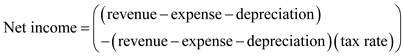…… (1)Substitute the respective values in Equation (1) to calculate the net income for the first year.Net income for the first year is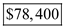.
Hence, option (c) is correct.

Tags
Choose question tagCompute the double-declining-balance depreciation schedule for an asset with the following data: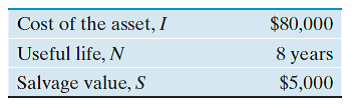Free
Essay

Given information:
• Cost of an asset is $80,000. • Salvage value is$5,000.
• Life time of the asset is 8 years.
The Double-declining-balance method of depreciation:
Depreciation value can be calculated by using the following formula of the double-declining-balance method.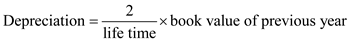…… (1)Book value:
Book value can be calculated by using the following formula.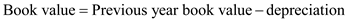…… (2)First year depreciation:
Substitute the respective values in equation (1) to calculate the first year depreciation.Depreciation in the first year is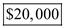.
Book value in the first year:
Substitute the respective values in equation (2) to calculate the first year book value.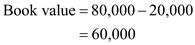First year book value is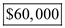.
Depreciation value and book value:
Hence, by using the Equation (1) and (2), Table-1 shows the depreciation value and book value based on the double-declining-balanced method.Table-1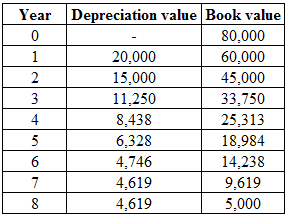Note: The depreciation value of double declining method is less than the straight line depreciation value from the year 7 to 8. Thus, there is a switch over the depreciation method from double declining method to straight line method from the year 7. The depreciation value from seventh year can be calculated as follows.Straight line depreciation value is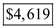.

Tags
Choose question tagNelson Company purchased equipment and incurred the following costs: Cash price = $55,000 Sales taxes =$4,400 Insurance during transit = $400 Site preparation, installation, and testing =$2,300 What amount should be used as the cost basis of the equipment?
Essay
Tags
Choose question tagA trucking company computes depreciation on its vehicles by a mileage basis. Suppose a delivery truck has a cost of $20,000, a salvage value of$2,000, and an estimated useful life of 200,000 miles. Determine the depreciation rate per mile. (a) $0.08 (b)$0.09 (c) $0.10 (d)$0.11
Multiple Choice
Tags
Choose question tagA builder paid $120,000 for a house and lot. The value of the land was appraised at$65,000, and the value of the house at $55,000. The house was then torn down at an additional cost of$8,000 so that a warehouse could be built on the lot at a cost of $50,000. What is the total value of the property with the warehouse? For depreciation purposes, what is the cost basis for the warehouse? Essay Answer: Tags Choose question tagCentronix Corporation purchased new equipment with an estimated useful life of five years. The cost of the equipment was$200,000, and the residual (salvage) value was estimated to be $25,000. In purchasing the new equipment, an old machine was traded in that had an original cost of$180,000, and had been depreciated at the rate of $18,000 a year. The trade-in allowance was$21,000, and accumulated depreciation amounted to $144,000 at the time of the exchange. What should be the cost basis of the new equipment for tax depreciation purposes? (a)$200,000 (b) $215,000 (c)$175,000 (d) $190,000 Multiple Choice Answer: Tags Choose question tagYou purchased a new molding machine for$155,000 by trading in a similar machine that had a book value of $18,000. Assuming that the trade-in allowance was$20,000 and that $85,000 cash was paid for the new asset, what is the cost basis of the new molding machine for depreciation purposes? Essay Answer: Tags Choose question tagA machine purchased for$45,000 has a depreciable life of four years. It will have an expected salvage value of $5,000 at the end of the depreciable life. Using the straight-line method, what is the book value at the end of year 2? (a)$27,500 (b) $20,000 (c)$35,000 (d) $25,000 Multiple Choice Answer: Tags Choose question tagConsider problem 9s.2. Suppose the salvage value at the end of year 4 is estimated to be$10,000 instead of $5,000. If the 200% method is used, what is the depreciation amount for year 3? a.$5,625 B) $10,000 C)$12,000 D) $18,000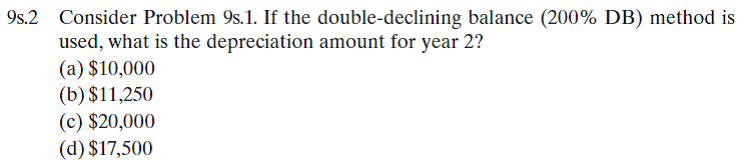Multiple Choice Answer: Tags Choose question tagIn Problem 9s.8, assume for the moment that (1) all sales were for cash and (2) all costs, except depreciation, were paid during year 1. How much cash would have been generated from operations? (a)$82,400 (b) $32,700 (c)$85,200 (d) $3,400 9s)8 Omar Shipping Company bought a tugboat for$75,000 (year 0) and expected to use it for five years after which it will be sold for $12,000. Suppose the company estimates the following revenues and expenses from the tugboat investment for the first operating year:If the company pays taxes at the rate of 30% on its taxable income, what is the net income during the first year? (a)$28,700 (b) $81,200 (c)$78,400 (d) $25,900 Multiple Choice Answer: Tags Choose question tagEl Dorado Machinery Company purchased a delivery truck at a cost of$32,000 on February 8, 2012. The truck has a useful life of six years with an estimated salvage value of $5,000. Compute the annual depreciation for the first two years using (a) The straight-line method. (b) The 150% declining-balance method. Essay Answer: Tags Choose question tagYou acquire a grinder priced at$65,000 by trading in a similar grinder and paying cash for the remaining balance. Assuming that the trade-in allowance is $11,000 and the book value of the asset traded in is$12,000, what is the cost basis of the new grinder for the computation of depreciation for tax purposes?
Essay
Tags
Choose question tagTo automate one of its production processes, Milwaukee Corporation bought three flexible manufacturing cells at a price of $400,000 each. When they were delivered, Milwaukee paid freight charges of$30,000 and handling fees of $15,000. Site preparation for these cells cost$50,000. Six employees, each earning $15 an hour, worked five 40-hour weeks to set up and test the manufacturing cells. Special wiring and other materials applicable to the new manufacturing cells cost$2,000. Determine the cost basis (the amount to be capitalized) for these cells.
Essay
Tags
Choose question tagSuppose that you placed a commercial building (warehouse) in service in January. The cost of property is $300,000, which includes the$100,000 value of land. Determine the amount of depreciation that is allowed during the first year of ownership. (a) $7,692 (b)$5,128 (c) $7,372 (d)$4,915
Multiple Choice
Tags
Choose question tagConsider the following data on an asset: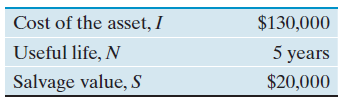Compute the annual depreciation allowance and the resulting book values using the following methods: (a) The straight-line depreciation method. (b) The double-declining-balance method.
Essay
Tags
Choose question tagConsider Problem 9s.1. If the double-declining balance (200% DB) method is used, what is the depreciation amount for year 2? (a) $10,000 (b)$11,250 (c) $20,000 (d)$17,500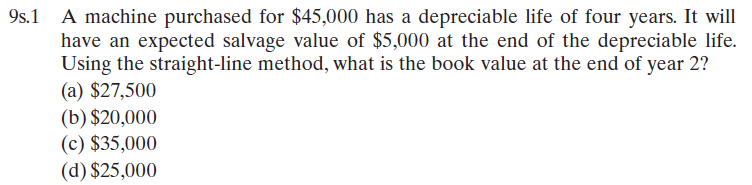Multiple Choice
Tags
Choose question tagIdentify which of the following expenditures is considered as a capital expenditure that must be capitalized (depreciated): (a) Purchase land to build a warehouse at $300,000. (b) Purchased a copy machine at$15,000. (c) Installed a conveyor system at a cost of $55,000 to automate some part of production processes. (d) Painted the office building, both interior and exterior, at a cost of$22,000. (e) Repaved the parking lot at a cost of $25,000. (f) Installed a purified water fountain in the employee lounge at a cost of$3,000. (g) Purchased a spare part for a stamping machine at a cost of $3,800. (h) Paid$12,000 to lease a dump truck for six months. (i) Purchased a patent on an energy-saving device over five years at a cost of $30,000. Essay Answer: Tags Choose question tagYou purchased a machine four years ago at a cost of$5,000. It has a book value of $1,300. It can be sold now for$2,300, or it could be used for three more years, at the end of which time it would have no salvage value. Assuming it is kept for three more years, what is the amount of economic loss during this ownership?
EssayMinolta Machine Shop just purchased a computer-controlled vertical drill press for $100,000. The drill press is classified as a three-year MACRS property. Minolta is planning to use the press for five years. Then Minolta will sell the press at the end of its service life for$20,000. The annual revenues are estimated to be $110,000. If the estimated net cash flow at the end of year 5 is$30,000, what are the estimated operating and maintenance expenses in year 5? Minolta's income tax rate is 40%. (a) $60,000 (b)$65,000 (c) $80,000 (d)$88,333